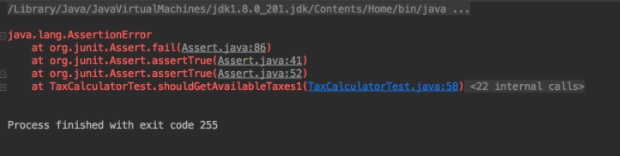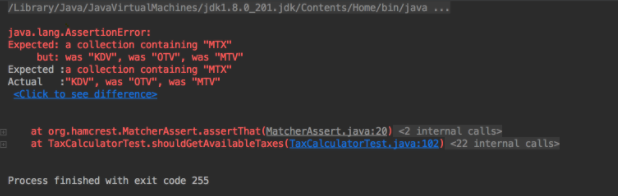Search:

# Better Unit Testing with Hamcrest

Category: java unittesting junit
Category: java unittesting junit

## Better Unit Testing with Hamcrest

Assertion methods are used to check whether the output of a method is what we expect. Junit provides us with a set of assertion methods like assertEquals, assertNotNull in Assert class. By using these static methods, we can assert various types like boolean and null. However, it is hard to use assertion methods of Junit because you may confuse expected with actual. We need better assertion matchers to write more readable and useful unit tests. Hamcrest framework offers nice matcher classes to do this. In this blog post, I will compare assertion methods of Junit and Hamcrest based on error messages and readability.

Before starting, you should add the XML code below to your pom.xml to use the methods of both frameworks. We exclude hamcrest-core in Junit because it does not include all the necessary methods. We need hamcrest-library to use all methods of Hamcrest.

``````
<dependency>
<groupId>junit</groupId>
<artifactId>junit</artifactId>
<version>4.12</version>
<scope>test</scope>
<exclusions>
<exclusion>
<groupId>org.hamcrest</groupId>
<artifactId>hamcrest-core</artifactId>
</exclusion>
</exclusions>
</dependency>

<dependency>
<groupId>org.hamcrest</groupId>
<artifactId>hamcrest-library</artifactId>
<version>1.3</version>
<scope>test</scope>
</dependency>
``````

Let’s create the TaxCalculator class as shown below. We will write unit tests for this class.

``````
public class TaxCalculator {
public static final BigDecimal DEFAULT_TAX_RATE = BigDecimal.valueOf(18);
public static final BigDecimal DEFAULT_DISCOUNT_RATE = BigDecimal.valueOf(10);
public static final List AVAILABLE_TAXES = Arrays.asList(“KDV”, “OTV”, “MTV”);

public BigDecimal calculate(BigDecimal amount, boolean hasDiscount) {
if (amount == null) {
return null;
}
if (!hasDiscount) {
return amount.multiply(DEFAULT_TAX_RATE)
.divide(BigDecimal.valueOf(100), 2, RoundingMode.HALF_UP);
}
BigDecimal effectiveRate = DEFAULT_TAX_RATE.subtract(DEFAULT_DISCOUNT_RATE);
BigDecimal tax = amount.multiply(effectiveRate)
.divide(BigDecimal.valueOf(100), 2,RoundingMode.HALF_UP);
return tax;
}
}
``````

Let’s create our first unit test for asserting the calculate method. assertEquals() is the method of Assert class in JUnit, assertThat() belongs to Matchers class of Hamcrest.

``````
@Test
public void shouldCalculateWithRounding() throws Exception {
BigDecimal actual = taxCalculator.calculate(BigDecimal.valueOf(10), false);
//JUnit assert
assertEquals(BigDecimal.valueOf(1.8).setScale(2, RoundingMode.HALF_UP), actual);

//Hamcrest matcher
assertThat(actual, is(equalTo(BigDecimal.valueOf(1.8).setScale(2, RoundingMode.HALF_UP))));
}
``````

Both methods assert the same thing; however, hamcrest matcher is more human-readable. As you see, it is like an English sentence “Assert that actual is equal to the expected value”. Let’s create a unit test for asserting available taxes.

``````
@Test
public void shouldGetAvailableTaxes() throws Exception {
List actual = TaxCalculator.AVAILABLE_TAXES;
//JUnit assertions
assertEquals(Arrays.asList(“KDV”, “OTV”, “MTV”), actual);
assertTrue(actual.contains(“KDV”));
assertTrue(!actual.contains(“KDM”));
assertEquals(3, actual.size());

//Hamcrest matcher
assertThat(actual, containsInAnyOrder(“KDV”, “OTV”, “MTV”));
assertThat(actual, not(contains(“KDV”, “MTV”, “OTV”)));
assertThat(actual, contains(“KDV”, “OTV”, “MTV”));
assertThat(actual, hasItems(“KDV”, “OTV”));
assertThat(actual, hasItem(“MTV”));
assertThat(actual, hasSize(3));
assertThat(actual, instanceOf(List.class));
assertThat(actual, everyItem(notNullValue(String.class)));
assertThat(actual, everyItem(not(isEmptyOrNullString())));
}
``````

Now, I will compare BigDecimal values by using both frameworks.

``````
@Test
public void shouldGetTaxRate() throws Exception {
BigDecimal actual = TaxCalculator.DEFAULT_TAX_RATE;
//JUnit assert
assertTrue(actual.compareTo(BigDecimal.valueOf(20)) < 0);
assertEquals(BigDecimal.valueOf(18), actual);

//Hamcrest matcher
assertThat(actual, closeTo(BigDecimal.valueOf(18), BigDecimal.valueOf(0)));
assertThat(actual, comparesEqualTo(BigDecimal.valueOf(18.000)));
assertThat(actual, is(lessThan(BigDecimal.valueOf(20))));
}
``````

For comparison, Junit does not offer special methods. Therefore, we should use the assertTrue method and add operators like < and > inside assertTrue. This looks ugly. With Hamcrest, we can compare actual and expected values using phrases similar to daily English sentences. “Assert that actual is less than the expected value.”

If you are interested in examples, you can find lists of all Assert methods by clicking the links at the end of the post.

When our tests fail, we need good error messages to understand what is going on. Let’s compare two error messages. The first one is from JUnit assertTrue method. The second one is from Hamcrest assertThat method. Both methods try to assert AVAILABLE_TAXES includes KDM.As you see, the error message of Hamcrest is more understandable. You can get why your test fails easily. However, the error message of assertTrue is hard to understand.

To sum up, we can get better error messages, and our code is more readable with Hamcrest. Therefore, I prefer to use it in my unit tests.